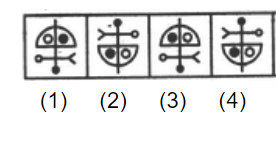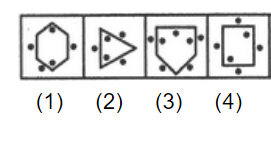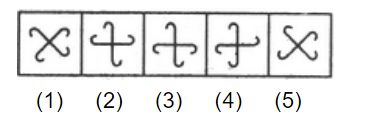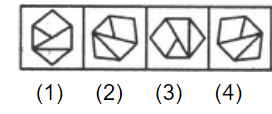# E-PolyLearning

 36. Choose the figure which is different from the rest.a. 1 b. 2 c. 3 d. 4

 37. Choose the figure which is different from the rest.a. 1 b. 2 c. 3 d. 4
 38. Choose the figure which is different from the rest.a. 1 b. 2 c. both 3 and 4 d. 5
 39. Choose the figure which is different from the rest.a. 1 b. 2 c. 3 d. 4
 40. Choose the figure which is different from the rest.a. 1 b. 2 c. 3 d. 4